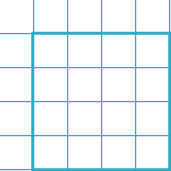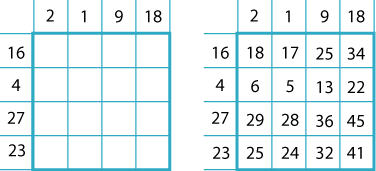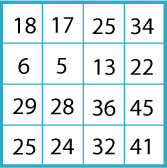#### You may also like### Prompt Cards

These two group activities use mathematical reasoning - one is numerical, one geometric.### Consecutive Numbers

An investigation involving adding and subtracting sets of consecutive numbers. Lots to find out, lots to explore.### Exploring Wild & Wonderful Number Patterns

EWWNP means Exploring Wild and Wonderful Number Patterns Created by Yourself! Investigate what happens if we create number patterns using some simple rules.

# Magic Matrix

##### Age 7 to 11Challenge Level
Here is a "magic" matrix: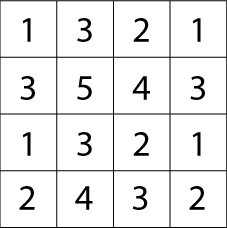It doesn't look very magical does it?
This is how you find out the "magic" in the matrix:

Circle any number in the matrix, for example, $5$. Draw a line through all the squares that lie in the same row and column as your selected number: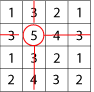Then circle another number that has not got a line through it, for example, the $1$ in the top right hand corner, and again cross out all squares in the same row and column: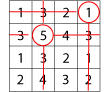Repeat for a third time, for example: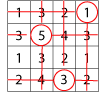Then circle only the remaining number that has no line through it: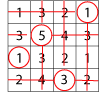Try again with a different starting number. What do you notice?

Try the same thing with these two slightly harder matrices: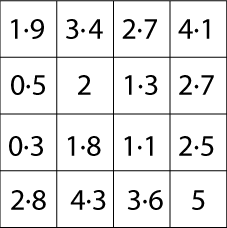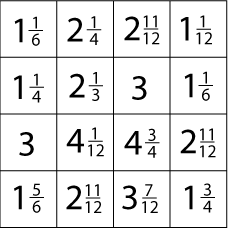This problem was made to celebrate NRICH's tenth birthday - perhaps you can see the connection!

Let's try a different one with larger numbers.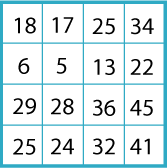What is the magic total this time?

I will show you how this kind of matrix works. You can then invent one to try on your friends!

First you need to choose your 'magic total'. As you know, I chose $100$ for the matrix above.
I have chosen: $1, 16, 9, 23, 18, 4, 2$ and $27$. [You can check that together they add to $100$.]

Now make an addition table like this: